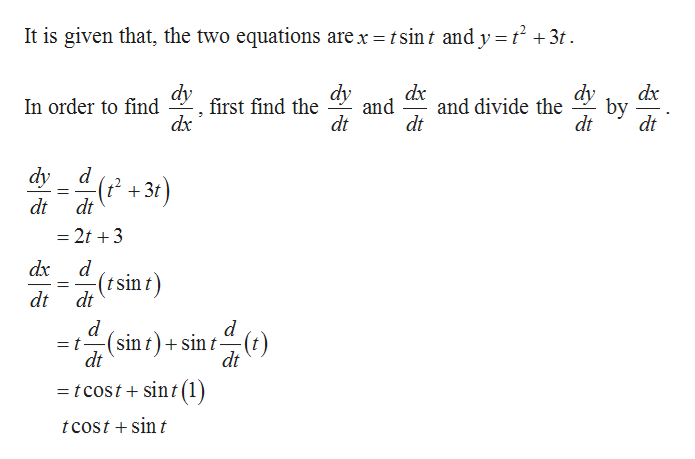Question
37 views

Find dy/dx.
x = t sin(t)
y = t2 + 3t

check_circle

Step 1help_outlineImage TranscriptioncloseIt is given that, the two equations are x tsint and y t2 +3t dy dx and and divide the dt dy dy by dt first find the dx In order to find dt dt dy d t+3t dt dt - 2t 3 d d(tsin t dt d d (sin t)sin t () dt = t dt = tcost + sint (1 tcost sin fullscreen

### Want to see the full answer?

See Solution

#### Want to see this answer and more?

Solutions are written by subject experts who are available 24/7. Questions are typically answered within 1 hour.*

See Solution
*Response times may vary by subject and question.
Tagged in

### Calculus